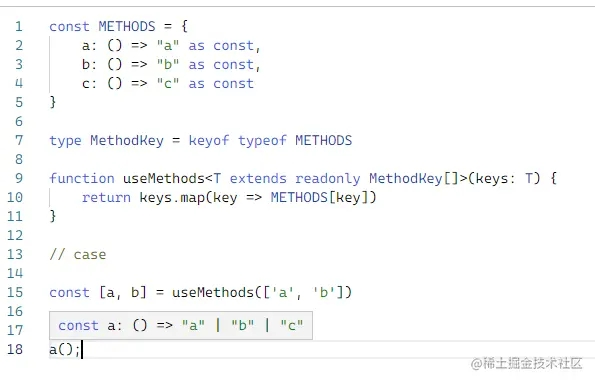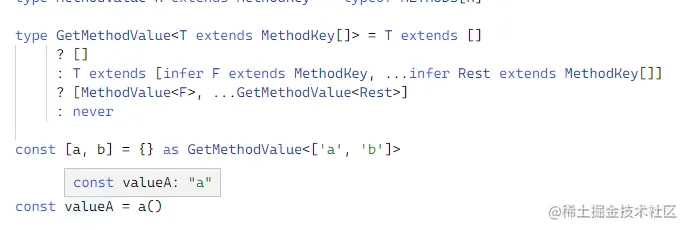# 簡單三行代碼函數實現幾十行Typescript類型推導

## 場景

```    const METHODS = {
a: () => "a" as const,
b: () => "b" as const,
c: () => "c" as const
}
```

```    type MethodKey = keyof typeof METHODS
function useMethods(keys: MethodKey[]) {
return keys.map(key => METHODS[key])
}
// case
const [a, b] = useMethods(['a', 'b'])
// expect to "a"
a();
```## 摸魚吃瓜式排查emmm……這還有些麻煩，先單獨寫個類型方法來推導結果試試，遞歸傳入的數組泛型，取出每一次的 `key` 對應的 `method`，再組合為數組。

``` type MethodValue<K extends MethodKey> = typeof METHODS[K]
type GetMethodValue<T extends MethodKey[]> = T extends []
? []
: T extends [infer F extends MethodKey, ...infer Rest extends MethodKey[]]
? [MethodValue<F>, ...GetMethodValue<Rest>]
: never
```## 元組大法

```type GetMethodValue<T extends (MethodKey | void)[]> = T extends []
? []
: T extends [infer F extends MethodKey, ...infer Rest extends MethodKey[]]
? [MethodValue<F>, ...GetMethodValue<Rest>]
: never
function useMethods<T extends ['a'?, 'b'?, 'c'?]>(keys: T) {
return keys.filter((key): key is MethodKey => !!key).map((key) => METHODS[key]) as GetMethodValue<T>
}
const [a, b] = useMethods(['a', 'b'])
const valueA = a()
``````    type Permutation<T, U = T> = [T] extends [never]
? []
: U extends T
? [U, ...Permutation<Exclude<T, U>>]
: never;
// expect to ['a', 'b'] | ['b', 'a']
type value = Permutation<'a' | 'b'>
```

```type Permutation<T, U = T> = [T] extends [never]
? []
: U extends T
? [U?, ...Permutation<Exclude<T, U>>]
: never;
type MethodKey = keyof typeof METHODS
type MethodValue<K extends MethodKey> = typeof METHODS[K]
type GetMethodValue<T extends (MethodKey | void)[]> = T extends []
? []
: T extends [infer F extends MethodKey, ...infer Rest extends MethodKey[]]
? [MethodValue<F>, ...GetMethodValue<Rest>]
: never
const METHODS = {
a: () => "a" as const,
b: () => "b" as const,
c: () => "c" as const
}
function useMethods<T extends Permutation<MethodKey>>(keys: T) {
return keys.filter((key): key is MethodKey => !!key)
.map((key) => METHODS[key]) as GetMethodValue<T>
}
const [a, b] = useMethods(['a', 'b'])
const valueA = a()
```